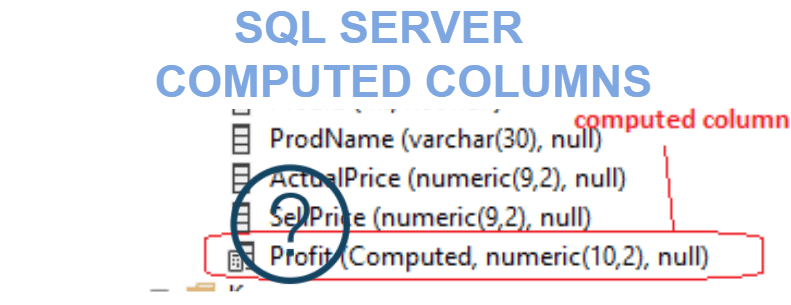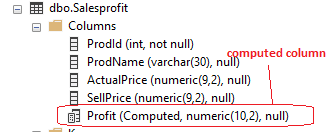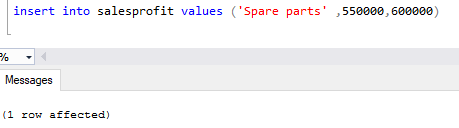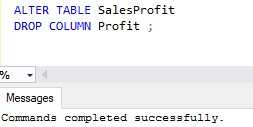Home » SQL Server Computed Columns

# SQL Server Computed ColumnsA computed column is a virtual column which is used to add a new column to a table with the value derived from the values of other columns in the same table.

Computed column is not physically stored in the table, unless column is marked PERSISTED, and it is persisted only if its expression is deterministic.

A computed column cannot be used as a DEFAULT or FOREIGN KEY constraint definition or with a NOT NULL constraint definition.

Lets take an example of Computed Column in SQL Server.

### Computed column when creating a table

First , we create a table named as Salesprofit and add computed column in table.

```Create table Salesprofit (

ProdId Int identity(1,1) ,

ProdName Varchar(30),

ActualPrice numeric(9,2) ,

SellPrice numeric(9,2) ,

Profit AS SellPrice - ActualPrice
)

```You can also check whether the computed column is created or not on table in object explorer.Lets insert a record into salesprofit table, SQL Server computes the value for the profit column based on the expression – SellPriceActualPrice

```insert into salesprofit values ('Spare parts' ,550000,600000)

```Lets select the records from salesProfit table.

```select * from Salesprofit

```As you can see , value for profit column is calculated by computed column automatically.

### Drop computed column from a table

you can also drop computed column from a table, using Alter table drop command

```ALTER TABLE SalesProfit

DROP COLUMN Profit ;

```Creating a persisted computed columns

You can also create a persisted computed columns, Data of the computed columns are physically stored on disk .

When data is changed in the table, SQL Server computes the result based on the expression of the computed columns and stores the results in these persisted columns physically.

You can make computed column persisted only if its expression is deterministic, It means that for any given set of inputs, computed expression always returns the same result set , it should not be changed again and again at certain time of periods.

For example: As we have used a expression for computed column profit – SellPriceActualPrice  that is deterministic.

If you use getdate() function in our expression than it will be a non deterministic expression as current date keeps changing and returns different value on different day.

```Alter table Salesprofit add salesdate as getdate() persisted

```As you can see, It gives an error of non-deterministic column.

If you remove persisted from query, then it will be created .

`Alter table Salesprofit add salesdate as getdate()  `As you have created a computed column on an existing table, so when you select records from a table it computes a salesdate.This site uses Akismet to reduce spam. Learn how your comment data is processed.